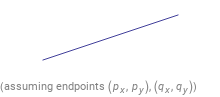Search IntMath
Close

# How to Draw a Line Segment in Geometry## What is a Line Segment?

A line segment is a part of a line that is bounded by two distinct end points and contains every point on the line between them. It is a basic concept in geometry and is used in many geometric proofs. A line segment can be represented by two points, which are labeled with letters.

In this article, we'll discuss how to draw a line segment. We'll cover the different methods used to draw a line segment as well as some practice problems to help you understand the concept better.

## Methods to Draw a Line Segment

There are several methods to draw a line segment. The most common way is to use a ruler or straight edge. To draw a line segment using a straight edge, you simply place the straight edge on the two points you want to connect and draw a line.

Another method to draw a line segment is to use a compass. To draw a line segment using a compass, you place the point of the compass on one of the points and draw an arc. Then, you place the point of the compass on the other point and draw another arc. Where the two arcs intersect is the line segment.

The last method to draw a line segment is to use a protractor. To draw a line segment using a protractor, you first measure the angle of the line segment. Then, you place the protractor so that its center is at one of the points. Finally, you draw an arc at the measured angle and the point where the arc intersects the protractor is the line segment.

## Practice Problems

Now, let's practice drawing line segments using the methods discussed above. The answers to the problems are at the end.

1. Use a straight edge to draw a line segment connecting the two points (-2,3) and (4,6).
2. Use a protractor to draw a line segment with an angle of 30 degrees connecting the two points (1,4) and (-2,2).
3. Use a compass to draw a line segment connecting the two points (5,0) and (-3,7).
4. Use a straight edge to draw a line segment connecting the two points (2,-3) and (-2,-7).
5. Use a protractor to draw a line segment with angle of 45 degrees connecting the two points (5,5) and (-5,-5).

## Summary

In this article, we discussed how to draw a line segment in geometry. We covered the different methods used to draw a line segment, such as using a ruler, a compass, and a protractor. We then practiced drawing line segments using each of these methods. Drawing a line segment is an important concept in geometry and one that should be mastered.

1. The line segment is (-2,3) to (4,6).
2. The line segment is (1,4) to (-2,2).
3. The line segment is (5,0) to (-3,7).
4. The line segment is (2,-3) to (-2,-7).
5. The line segment is (5,5) to (-5,-5).

## FAQ

### How is a line segment defined?

A line segment is a straight line that connects two points. It has a definite length but no width. It is the shortest distance between two points.

### What are the two methods of constructing a line segment?

The two methods of constructing a line segment are using a ruler and using a compass. With a ruler, you can measure out the desired length and mark two points on the line. With a compass, you can draw a circle with a given radius and mark two points on the circumference of the circle.

## Problem SolverThis tool combines the power of mathematical computation engine that excels at solving mathematical formulas with the power of GPT large language models to parse and generate natural language. This creates math problem solver thats more accurate than ChatGPT, more flexible than a calculator, and faster answers than a human tutor. Learn More.